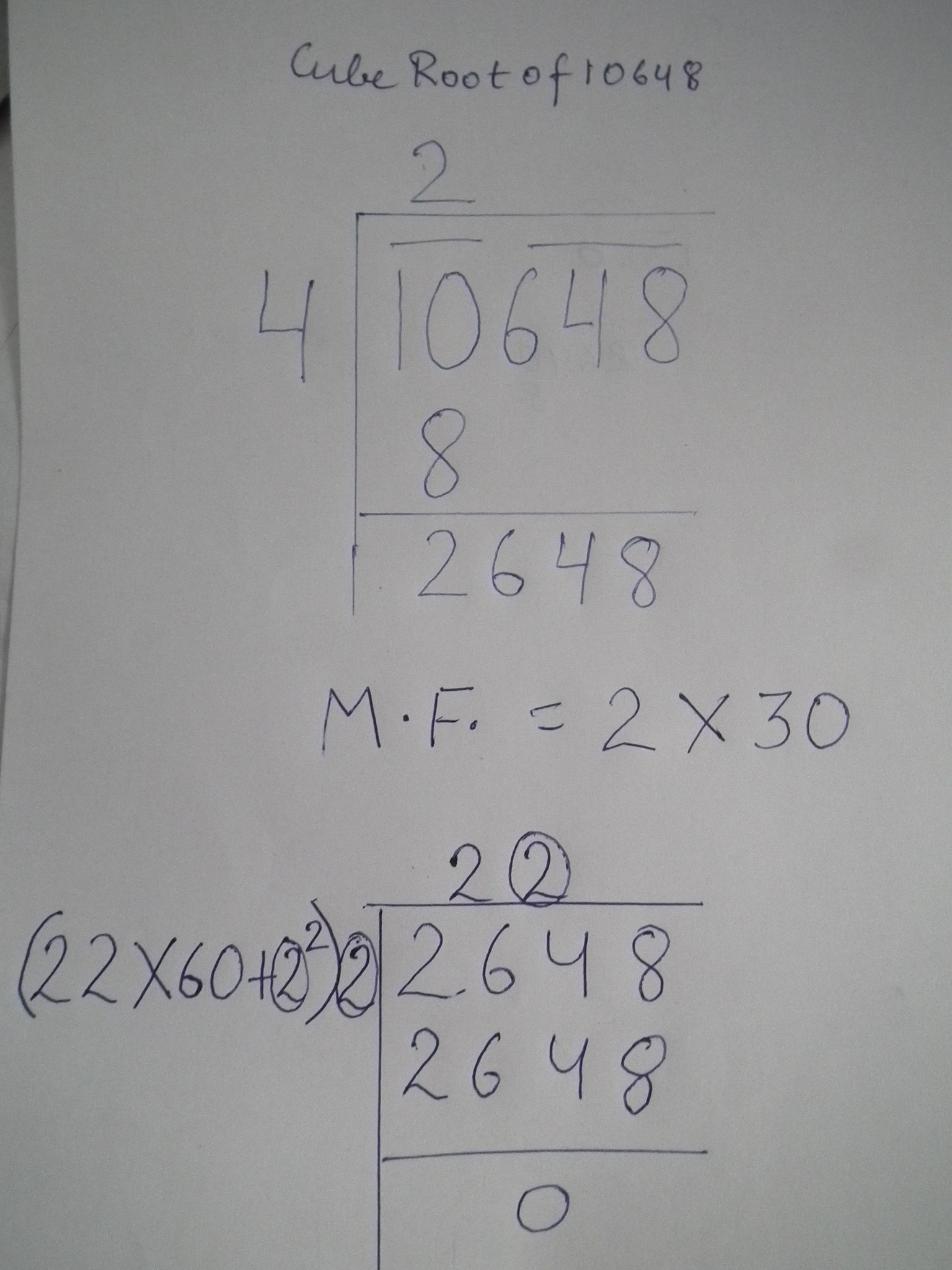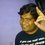# Long divison method of cube rootlong divison method for finding cube root

for example lets take cube of 21 i.e. 9261.

now make make pair of 3 from back i.e 9 and 261

find the no. whose cube root is less than or equal to 9 . obviously its 2

subtract 8 from 9 and write down the 2nd pair i.e 261 as in square root method. we have now 1261

now we have to find the multiplication factor for further process that comes by multiplying the first obtained digit i.e 2 by 30 i.e 60

now we have to find 2nd digit . the second digit is such that when the no. formed by 1 and 2nd digit multiplied by multiplication factor is added to square of the digit is multiplied by 2nd digit the result should be less than or equal to 1261.

like in this case we by choosing 1 we get above no. 21 and adding 21*60 + 1 i.e square of 1 we get 1261 and 1261 multiplied by 1 i.e 2nd digit of required no. 1261 is the result and subtracting 1261 from 1261 we get remainder 0 i.e 21 is the cube root of 9261

lets take more complex example

cube of 463 is 99252847

now first make pair of 3 from backwards i.e 99, 252, 847

64 is less than 99 so 4 is our first digit

write down 99-64= 35 suffixed with 252 i.e 35252

multiplication factor will be 30 *4=120

choosing second digit 6 gives us 46*120+36(36 being square of 6)=5556 and 5556 multiplied by 6 gives 33336....

again subtract 35252 and 33336 and suffix 3rd pair i.e 1916847

now our multiplication factor will be 46*30= 1380

choosing 3 as 3rd digit will give us 463*1380+9(square of 3) = 638949 which multiplied by 3rd digit i.e 3 gives 1916847

our remainder as we can see is zero so the cube root of 99252847 is 463Note by Anshul Gupta
6 years, 5 months ago

This discussion board is a place to discuss our Daily Challenges and the math and science related to those challenges. Explanations are more than just a solution — they should explain the steps and thinking strategies that you used to obtain the solution. Comments should further the discussion of math and science.

When posting on Brilliant:

• Use the emojis to react to an explanation, whether you're congratulating a job well done , or just really confused .
• Ask specific questions about the challenge or the steps in somebody's explanation. Well-posed questions can add a lot to the discussion, but posting "I don't understand!" doesn't help anyone.
• Try to contribute something new to the discussion, whether it is an extension, generalization or other idea related to the challenge.

MarkdownAppears as
*italics* or _italics_ italics
**bold** or __bold__ bold
- bulleted- list
• bulleted
• list
1. numbered2. list
1. numbered
2. list
Note: you must add a full line of space before and after lists for them to show up correctly
paragraph 1paragraph 2

paragraph 1

paragraph 2

[example link](https://brilliant.org)example link
> This is a quote
This is a quote
    # I indented these lines
# 4 spaces, and now they show
# up as a code block.

print "hello world"
# I indented these lines
# 4 spaces, and now they show
# up as a code block.

print "hello world"
MathAppears as
Remember to wrap math in $$ ... $$ or $ ... $ to ensure proper formatting.
2 \times 3 $2 \times 3$
2^{34} $2^{34}$
a_{i-1} $a_{i-1}$
\frac{2}{3} $\frac{2}{3}$
\sqrt{2} $\sqrt{2}$
\sum_{i=1}^3 $\sum_{i=1}^3$
\sin \theta $\sin \theta$
\boxed{123} $\boxed{123}$